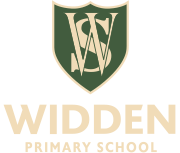Skip to content ↓# Maths

Maths is taught across the school following the White Rose schemes of work.  We are currently developing a mastery approach to the teaching of maths.  All maths teaching follows the concrete to pictorial to abstract approach.  All classes are full of counters, beads, cubes and mathematical talk!

Here is a link to the White Rose schemes of work that we base our planning on:

https://whiterosemaths.com/resources/schemes-of-learning/primary-sols/

Here is our maths Calculation Policy which shows you the order in which we teach the four calculations (addition, subtraction, multiplication and division) and how we do this.